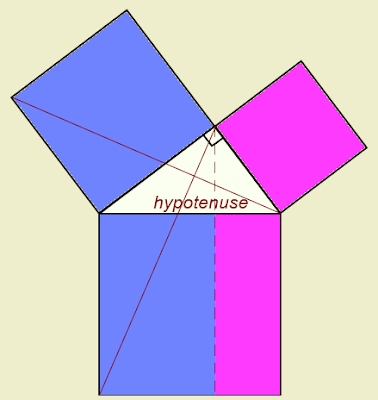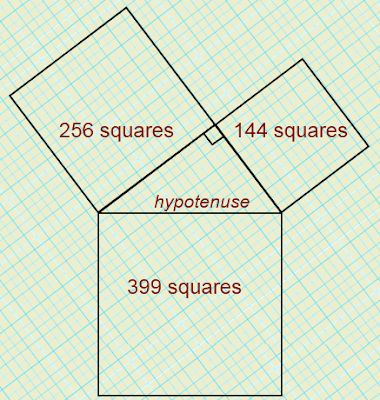## Friday, January 15, 2010

### On the HypotenuseThe area of the square on the hypotenuse of any right-angle triangle
is equal to the sum of the areas of the squares on the other sides.
Pythagoras' Theorem

I was hopeless at Mathematics when I began High School. Teachers despaired at my ineptitude. But I had a natural interest in Science.

It was mainly due to good Scottish teaching, and a genuine willingness on my part to engage in learning, that I studied Mathematics to a level that let me reach the highest academic levels in Science.

It was also due in part to the curriculum I followed. I believe that it taught me how to think. It wasn’t that it taught me to think. I could do that. It taught me how to think, and to be able to think in specific ways.

In the 1970s, I taught High School Mathematics to senior level.

Recently, I have become familiar with fundamental changes in the way mathematical thinking is taught in High School in New Zealand.
I suspect that these are similar to changes in the Mathematics curricula in other western countries.

Get it right

The Euclidean proof of the useful Theorem of Pythagoras was one of the things I had to learn for my early qualifying examinations at High School. My mother learnt the same proof when she attended High School.

Euclid’s proof was taught in New Zealand schools until changes were made in the curriculum towards the end of last century (
20th). It is now supplanted by what I’d call a non-proof. What young minds are required to study about the Pythagorean Theorem in New Zealand today is certainly not a proof of it.

Euclid’s proof is based on the properties of rectangles and of triangles that are each of the same shape and size – as well as associated geometry ideas involving these shapes drawn between parallel lines. There are several hundred proofs of this fundamental theorem. Some are outlined in Wikipedia. A few I learnt at High School.

All of the proofs I learnt permitted me to understand, not only how the Pythagorean Theorem can be proved, but also what it is to know the significance of a universal proof. It is a way of thinking that permits a learner to appreciate that a proof needs to be watertight.

What does it prove?

Euclid’s proof can be applied to ANY right-angle triangle, not just a few special triangles. Therein is the difference between what used to be taught and what is now taught.

In High School today, a learner in Mathematics is instructed on how to show that a given right-angle triangle has the Pythagorean property. The instruction is not about any right-angle triangle, but applies only to a particular right-angle triangle that the learner draws.

Essentially, learners follow a recipe that shows that the Theorem may work for their triangle. It provides no real understanding of proof.

Here’s what they are instructed to do:Learners in Mathematics are shown how to draw a right-angle triangle on a grid, using simple mathematical drawing equipment. They are also shown how to draw squares on each of the three sides of the triangle. By counting the number of grid squares that make up each of the squares, they can show that the Theorem is followed approximately.

Higher thinking

While this recipe permits the learner to practice skills in using drawing equipment, it provides no understanding of a mathematical proof.
It does not even show conclusively that the Theorem works.
However carefully it is done, not every attempt at adding the squares will show that the Theorem is precise. Check out the squares shown above.

One can argue over the need for the knowledge of how to prove the Pythagorean Theorem. But the significant learning is nothing to do with that knowledge. The thinking skills learnt that permit the learner to understand what a watertight proof is all about are really what are far more useful and relevant to higher thinking skills.

This is the whole point of teaching the proof. It has the potential to permit the learner to realise the significance of a theorem that can be applied universally, and why it has this property. It is a way of thinking analytically that is not being taught today.